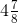# Fractions and Decimals Chapter 2 Class 7 Maths NCERT PDF

‘NCERT Solutions for Class 7 Maths Chapter 2′ PDF Quick download link is given at the bottom of this article. You can see the PDF demo, size of the PDF, page numbers, and direct download Free PDF of ‘Ncert Class 7 Maths Chapter 2 Exercise Solution’ using the download button.

### Chapter 2: Fractions and Decimals Textbook With Solutions

#### 2.1 INTRODUCTION

You have learned fractions and decimals in earlier classes. The study of fractions included proper, improper, and mixed fractions as well as their addition and subtraction.

We also studied the comparison of fractions, equivalent fractions, representation of fractions on the number line, and ordering of fractions.

Our study of decimals included their comparison, their representation on the number line, and their addition and subtraction. We shall now learn multiplication and division of fractions as well as decimals.

#### 2.2 HOW WELL HAVE YOU LEARNT ABOUT FRACTIONS?

A proper fraction is a fraction that represents a part of a whole.

Is 7/4 a proper fraction? Which is bigger, the numerator or the denominator?

An improper fraction is a combination of whole and a proper fraction.

Is 7/4 an improper fraction? Which is bigger here, the numerator or the denominator?
The improper fraction 7/4 can be written as

### NCERT Solutions Class 7 Maths Chapter 2 Fractions and Decimals

Exercise 2.1 Page: 31 Solution

1. Solve:

(i) 2 – (3/5)

Solution:-

For subtraction of two unlike fractions, first change them to the like fractions.

LCM of 1, 5 = 5

Now, let us change each of the given fraction into an equivalent fraction having 5 as the denominator.

= [(2/1) × (5/5)] = (10/5)

= [(3/5) × (1/1)] = (3/5)

Now,

= (10/5) – (3/5)

= [(10 – 3)/5]

= (7/5)

(ii) 4 + (7/8)

Solution:-

For addition of two unlike fractions, first change them to the like fractions.

LCM of 1, 8 = 8

Now, let us change each of the given fraction into an equivalent fraction having 8 as the denominator.

= [(4/1) × (8/8)] = (32/8)

= [(7/8) × (1/1)] = (7/8)

Now,

= (32/8) + (7/8)

= [(32 + 7)/8]

= (39/8)

=